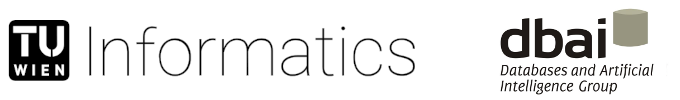Path: DBAI > Research > Projects > D-FLAT Project > Software > D-FLAT System > Practice > Maximum Independent Set

Tools: Print

# Maximum Independent Set

``````
%dflat: --tables -e vertex -e edge -d td -n semi --no-empty-leaves
%add this to command or modeline: --no-empty-root

% Extend table rows from child node.
1 { extend(R) : childRow(R,N) } 1 :- childNode(N).

% Only join rows that conincide on vertices belonging to the set.
:- extend(R;S), childItem(R,in(X)), not childItem(S,in(X)).

% Retain info as to which vertices are in the set.
item(in(X)) :- extend(R), childItem(R,in(X)), not removed(X).

% Guess vertices for the independent set.
{ item(in(X)) : introduced(X) }.

% No adjacent vertices must exist in the set.
:- edge(X,Y), item(in(X);in(Y)).

% We use negative costs since this is a maximization problem and D-FLAT
% only minimizes.
% Leaf costs
cost(-C) :- initial, C = #count{ X : item(in(X)) }.
% Exchange costs
cost(CC - IC) :- numChildNodes == 1, extend(R), childCost(R,CC),
IC = #count{ X : item(in(X)), introduced(X) }.
% Join costs
cost(C1 + C2 - CC) :- numChildNodes == 2, extend(R1;R2),
childCost(R1,C1;R2,C2), commonCost(R1,R2,CC).
commonCost(R1,R2,-CC) :- childRow(R1,N1;R2,N2), N1 < N2,
CC = #count { X : childItem(R1,in(X);R2,in(X)) }.

#show item/1. #show cost/1. #show extend/1.

``````
Last updated: 2017-08-30 17:52

Home / Kontakt / Webmaster / Offenlegung gemäß § 25 Mediengesetz: Inhaber der Website ist das Institut für Logic and Computation an der Technischen Universität Wien, 1040 Wien. Die TU Wien distanziert sich von den Inhalten aller extern gelinkten Seiten und übernimmt diesbezüglich keine Haftung. Disclaimer / Datenschutzerklärung